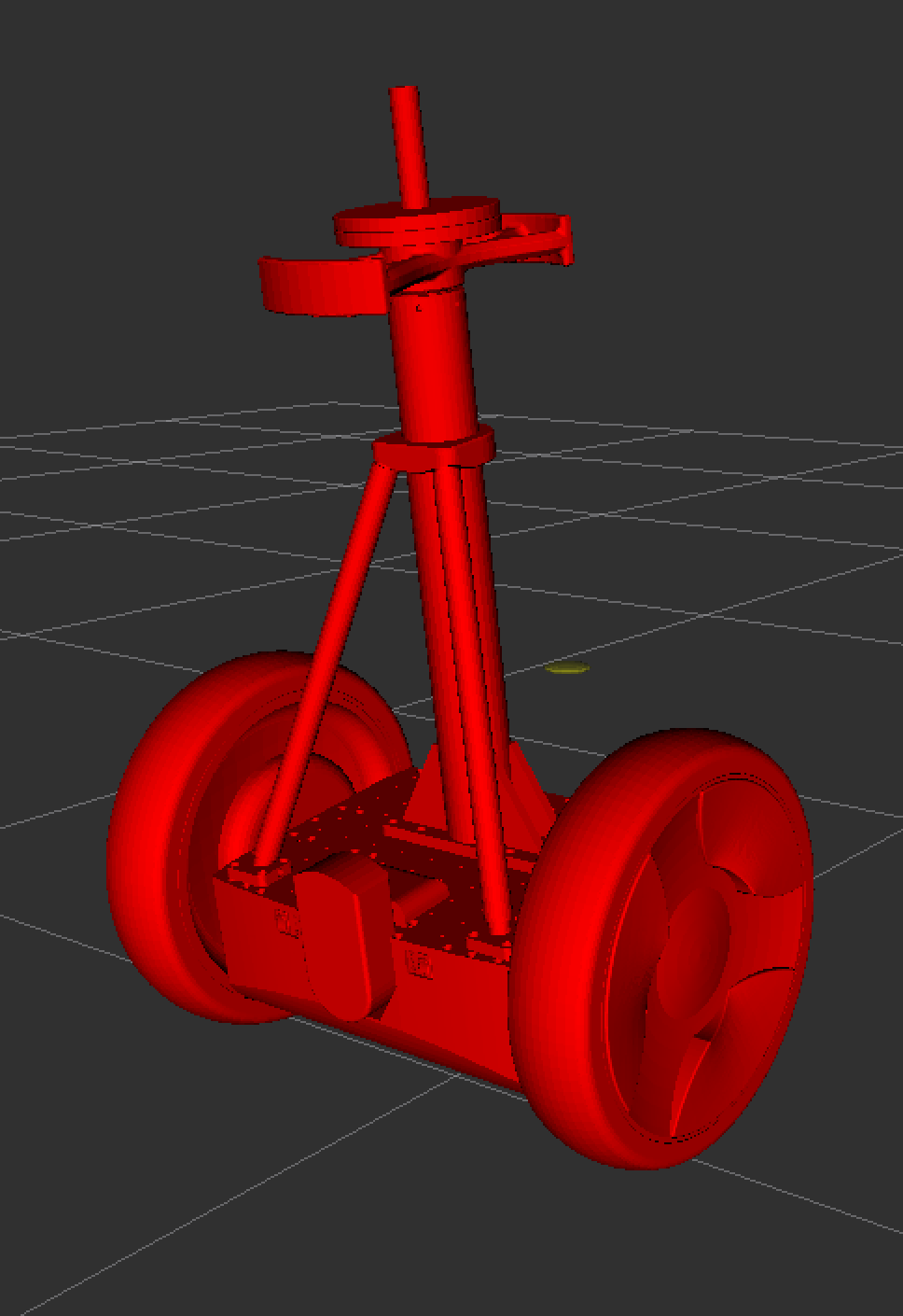# LCSS 2022: A Constructive Method for Designing Safe Multirate Controllers for Differentially-Flat Systems

Devansh R. Agrawal*, Hardik Parwana, Ryan K. Cosner*, Ugo Rosolia, Aaron D. Ames, Dmitra Panagou.

Abstract: We present a multi-rate control architecture that leverages fundamental properties of differential flatness to synthesize controllers for safety-critical nonlinear dynamical systems subject to input constraints. We propose a two-layer architecture, where the high-level generates reference trajectories using a linear Model Predictive Controller, and the low-level tracks this reference using a feedback controller. The novelty lies in how we couple these layers, to achieve formal guarantees on recursive feasibility of the MPC problem, and safety of the nonlinear system. Furthermore, using differential-flatness, we provide a constructive means to synthesize the multi-rate controller, thereby removing the need to search for suitable Lyapunov or barrier functions, or to approximately linearize/discretize nonlinear dynamics. We show the synthesized controller is a convex optimization problem, making it amenable to real-time implementations. The method is demonstrated experimentally on a ground rover and a quadruped robotic system.

This is work performed in collaboration with Sarah Dean and Ben Recht (UC Berkeley) and Andrew Taylor and Aaron Ames (Caltech). It was originally published at the 2020 Conference on Robotic Learning. The extended publication can be found here.

Below is a simplified version of the paper meant for a general audience. (This one’s for you mom!)

# Introduction

Safety is really important, but most of our methods of assuring safety really on highly accurate system measurements. We explored the problem of ensuring safety despite the measurement errors. We apply our solution to a simulated segway system with a camera-in-the-loop sensor.

# Background

When designing a system we often require that the final design is “safe”. But what exactly does it mean to be safe? For our purposes we define a safe set as a region of space where our system is considered safe. Like an autonomous vehicle is safe if it’s one the road and unsafe if it’s not on the road. More precisely, we say that a system is safe if that safe set is invariant.

Safety (Invariance): A system is safe if starting in the safe set implies that it will stay in the safe set.

To ensure this type of safety we consider the dynamics of the system:

$$\dot{\mathbf{x}} = \mathbf{f}(\mathbf{x}) + \mathbf{g}(\mathbf{x})\mathbf{u}$$

and a safe set defined as a 0-superlevel set of a continuously differentiable safety function $$h$$ (aka places where $$h(\mathbf{x})>0$$).

This function $$h$$ is a Control Barrier Function if there exists inputs such that:

$$\dot{h}( \mathbf{x}, \mathbf{u}) \geq - \alpha(h(\mathbf{x})).$$

When this inequality is ensured, the system is guaranteed to be safe (Thrm 1).

# New Theory: Measurement-Robust Control Barrier Functions

Often when working with real systems we can’t measure things exactly, but Control Barrier Functions incorporate the current value of $$\mathbf{x}$$. In this work we found a way of extending existing CBF theory to ensure that the system remains safe even despite thesse measurement errors.

The CBF $$h$$ is Measurement Robust if there exists a controller that satisfies the constraint:

$$\dot{h}(\hat{\mathbf{x}},\mathbf{u}) - \epsilon (\mathfrak{L}_{L_fh} + \mathfrak{L}_{\alpha \circ h} + \mathfrak{L}_{L_gh} ||\mathbf{u}||_2) \geq - \alpha ( h (\hat{\mathbf{x}}))$$

The difference between this Meausrement Robust CBF condition and the standard CBF condition is the addition of the term:

$$-\epsilon (\mathfrak{L}_{L_fh} + \mathfrak{L}_{\alpha \circ h} + \mathfrak{L}_{L_gh} ||\mathbf{u}||_2)$$

Here $$\epsilon$$ is represents how bad our measurement is and the $$\mathfrak{L}$$ terms represent how “smooth” our dynamics are. Intuitively, if the dynamics are really “smooth” then small measurement errors will have little effect, but if the dynamics aren’t smooth and change really quickly then small measurement errors can result in large mispredictions of the system’s behavior.

# Learning for Measurement Model Uncertainty Reduction

Because the input, $$\mathbf{u}$$, appears in this smoothed term, it is possible that no inputs exist that ensure safety. In particular, our formulation implies that if the following condition does not hold, then it is impossible to render the system safe:

$$\epsilon \leq \textrm{max}\left\{ \frac{||L_gh(\hat{\mathbf{x})}||_2}{\mathfrak{L}_{L_gh}}, \frac{L_fh(\hat{\mathbf{x}}) + \alpha(h(\hat{mathbf{x}}))}{\mathfrak{L_fh} + \mathfrak{L}_{\alpha\circ h}} \right\}$$.

In the context of machine learning, this inequality suggests the use of a sampling scheme that will ensure that the measurement error never reaches an unsafe level.

# Simulation Results

Due to Covid19 restrictions, the experiments for this work were performed exclusively in simulation. They were performed using on a simulated Segway. The system was considered safe as long as it remained upright within a certain angle window.Two types of errors were considered: the worst case error synthetically added to the measurement and the error found when measuring the system using a learned model which estimated the position based on camera data.

In both cases the standard CBF controller failed to ensure safety and the MRCBF controller succeeded.